## Automated Simple Counter using Microsoft Excel

In this tutorial, you will learn step by step how to use MS Excel iteration with a working example to make simple counter. Excel iteration is a great tool to perform many interactive simple programs in MS Excel without VBA macro. See how it works in this tutorial.

Why use iteration instead of VBA macro?
How to use iteration in MS Excel?
Working Example of MS Excel iteration

Why use iteration instead of VBA macro?

Some MS Excel users annoyed by the warning of the existing macro like this: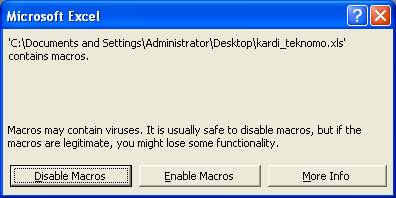They may disable the macro but the program will not work. They are never sure whether the worksheet contains viruses or not. This is quite big dilemma for people who do not like to take risk.

Is there any way to make a program in Excel with iteration (similar to While Loop or For Next) without Macro?

Yes, MS Excel iteration can do great job of For-Next Loop for you with no programming at all (actually you may need programming in spreadsheet, but without the macro). Using Microsoft Excel, you can iterate without VBA macro. Thus, your users do not need to worry about the annoying warning about viruses. This MS Excel iteration is one of great tool if you know how to use circular reference for your benefit

How to use iteration in MS Excel?

The key idea on how to use MS excel iteration is to make a circular link in the spreadsheet to accumulate the value and check the Iteration option in menu Tools-Options-Calculation Tab Iteration check box.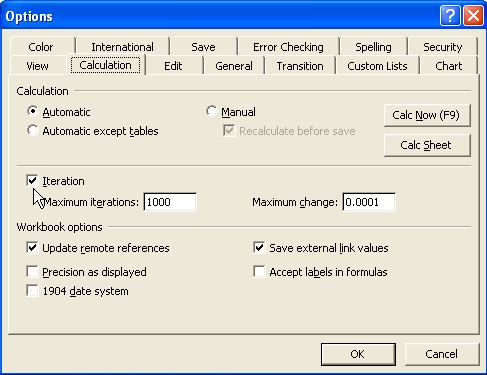Microsoft excel halt the calculation after Maximum iteration (default is 1000) or after all values in the formulas change by less than Maximum Change (default is 0.0001) between iterations. The loop is automatically bounded either by maximum iterations OR by maximum change between two consecutive iterations. To run the iteration again, you need to press F9.

If you put the Calculation option as Automatic , all computation will be done as usual. If you use Calculation option as Manual, you need to press F9 every step to make the computation change.

Working Example of MS Excel iteration

As an example of MS iteration, let us build a simple counter program. Here is the procedure step by step

1. Type 0 in B3, and 100 in B4. Type Start in A3, End in A4 and Counter in A5.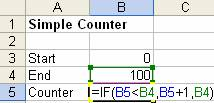2. In B5, type =IF(B5<B4, B5+1, B4). Its mean if the counter below 100, Ignore the warning of circular reference by click OK to message below.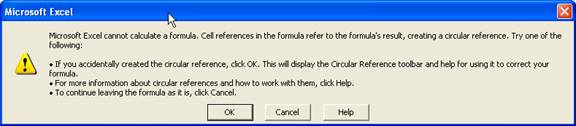3. Click menu Tools > Options > Calculation Tab. Set the calculation option to Manual , check the Iteration check box and set the Maximum iteration to 1 (see figure below)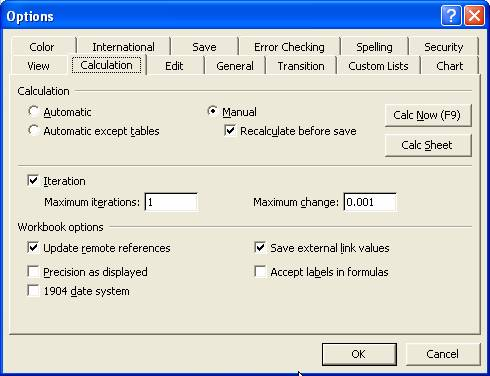4. The simple counter starts with 1. Press F9 function key to increase the counter one at a time until 100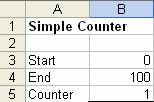The iteration works very well and the counter can do the job manually. Every time user needs to increase the counter, he or she needs to press F9. To press F9 100 times manually, however, is not a good job for our users. Can we automate the iteration? Yes, you can automate the iteration by setting Calculation option to Automatic and maximum iteration to 100 or 1000 .

This counter, however, still have one problem. If you want to change the initial value of the iteration, you need to type the formula again every time you want to compute. Otherwise, it will use the computed value as the initial value. Some initial value may produce unstable result and #NUM as error in computation. It is quite annoying to retype (or paste) manually the formula again to correct the mistake.

How do we restart the counter back to zero and avoid those initial value problems?

1. Continue our counter above, Delete to Start in D3. Put special color to the font and cell to distinguish it as a control button

2. Type additional If-Then rule in B5 becomes =IF(D3="",B3, IF(B5<B4,B5+1,B4) )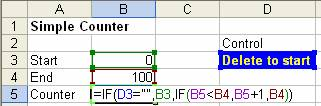3. Click menu Tools > Options > Calculation Tab. Set the calculation option to Automatic, check on the Iteration check box and set the Maximum iteration to 1000 (see figure below)4. Every time user deletes the content of control cell D3, the counter reset to zero. If user type any letter or number in the control cell D3, the counter automatically run until reach maximum value of 100. (You may change the value of B4 to 500 and see what happen)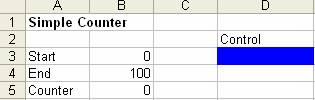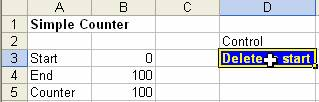Now you have completed the simple counter with control to reset the counter.

Special note for scientific procedures : Unless you are sure, the convergence of the iteration, the results of the some iteration may be misleading. What you get as the result is the value after Maximum iteration. Another weakness of iteration: You cannot graph the value of the iteration process (use ordinary spreadsheet to do this). For example, if there is a cycle you may not see clearly whether the iteration is convergence.

Preferable reference for this tutorial is

Teknomo, Kardi (2015) How to use MS Excel Iteration. https:\\people.revoledu.com\kardi\ tutorial\Excel\Iteration.html